# Steady State AC Magnetic: solved equations (vector model 2D)

## Introduction

The vector model is the general model proposed for 2D applications.

## Equation solved with vector model (2D applications)

The Maxwell-Faraday equation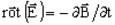implies presence of electric scalar potential V, such as: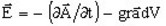The equation solved by the finite elements method in a Steady State AC Magnetic application is written: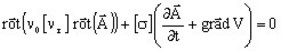The complex image of this equation (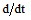is replaced by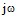) is the following equation: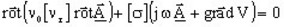where:

• r] is the tensor of the reluctivity of the medium
• ν0 is the reluctivity of the vacuum; ν0 = 1/μ0 = 1/(4 π 10-7) (in m/H)
•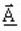is the magnetic vector complex potential (in Wb/m)
• [σ] is the tensor of the conductivity of the medium (in S)
• V is the electric scalar potential (in V)

## State variables, vector model (2D)

The state variables are:

• the magnetic vector complex potential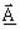• the electric scalar potential V

The state variables, dependent on the problem type, plane 2D or axisymmetric 2D, are given in the table below.

Type of the problem State variable

Notation (in Flux 3D)

plane An AN2*
axisymmetric r.An RAN2
Note: * The index 2 signifies that the quantity is a complex one.